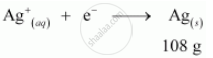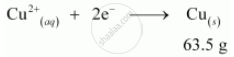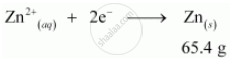Share

# Three electrolytic cells A,B,C containing solutions of ZnSO4, AgNO3 and CuSO4, respectively are connected in series. A steady current of 1.5 amperes was passed through them until 1.45 g of silver deposited at the cathode of cell B - CBSE (Science) Class 12 - Chemistry

ConceptElectrolytic Cells and Electrolysis - Introduction

#### Question

Three electrolytic cells A,B,C containing solutions of ZnSO4, AgNO3 and CuSO4, respectively are connected in series. A steady current of 1.5 amperes was passed through them until 1.45 g of silver deposited at the cathode of cell B. How long did the current flow? What mass of copper and zinc were deposited?

#### Solution

According to the reaction:i.e., 108 g of Ag is deposited by 96487 C.

Therefore, 1.45 g of Ag is deposited by  = (96487xx1.45)/108 C

= 1295.43 C

Given,

Current = 1.5 A

∴ Time = (1295.43)/1.5s

= 863.6 s

= 864 s

= 14.40 min

Again,i.e., 2 × 96487 C of charge deposit = 63.5 g of Cu

Therefore, 1295.43 C of charge will deposit = (63.5xx1295.43)/(2xx96487)g

= 0.426 g of Cui.e., 2 × 96487 C of charge deposit = 65.4 g of Zn

Therefore, 1295.43 C of charge will deposit  = (65.4xx1295.43)/(2xx96487)g

= 0.439 g of Zn

Is there an error in this question or solution?

#### Video TutorialsVIEW ALL 

Solution Three electrolytic cells A,B,C containing solutions of ZnSO4, AgNO3 and CuSO4, respectively are connected in series. A steady current of 1.5 amperes was passed through them until 1.45 g of silver deposited at the cathode of cell B Concept: Electrolytic Cells and Electrolysis - Introduction.
S